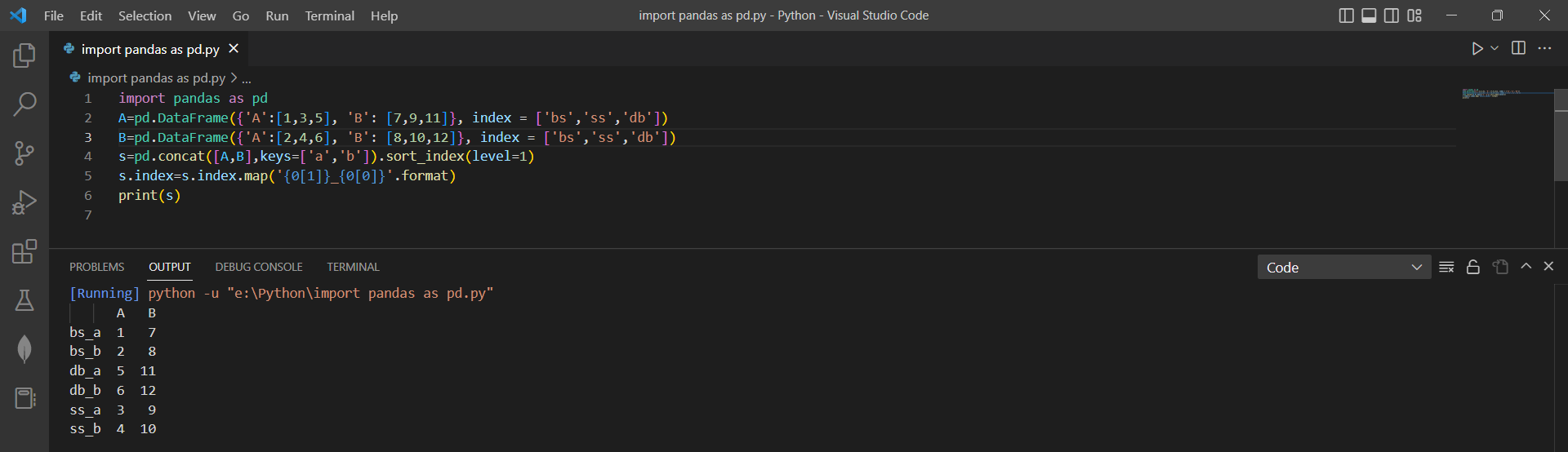# Concatenating Pandas DataFramesby vigneshchennai74Updated: Feb 20, 2023Solution Kit

The concat function is used to perform concatenation in Pandas. In Pandas, we can concatenate dataframes vertically or horizontally. Vertical concatenation stacks dataframes on each other, while horizontal concatenation concatenates dataframes side-by-side.

Concatenating Pandas DataFrames can be helpful in several ways, such as:

• Combining data from multiple sources: If we have data stored in multiple dataframes, we can use concatenation to combine the data into a single dataframe for analysis or visualization.
• Adding missing data: If we have missing data in a dataframe, we can add the missing data from another dataframe using concatenation.
• Adding metadata: We can add a hierarchical index to the concatenated dataframe to identify the source of each row. This can be useful when we are combining data from multiple sources.
• Data analysis: Once we have concatenated the dataframes, we can perform any necessary data cleaning or analysis on the resulting dataframe. We can also use slicing or filtering to extract subsets of the data based on the index values.

pd.concat() is a Python pandas library function that lets you concatenate two or more dataframes along a particular axis. The function takes a sequence of dataframes as input and returns a new concatenated dataframe. Concatenating Pandas DataFrames is combining two or more dataframes into a single dataframe. This can be useful when we have data in separate dataframes that we want to combine for analysis, visualization, or export.

Overall, concatenating Pandas DataFrames can be a powerful tool for managing and analyzing data, especially when dealing with large datasets or data stored in multiple sources.

Here is an example of how to concante pandas Dataframe:Preview of the output that you will get on running this code from your IDE

### Code

In this solution we have used Pandas Library.

1. Copy this code using "copy " button above and paste it in your Python IDE.
2. Import Pandas Library into the code.
3. Enter the Dataframe (df) which user need to merge
4. Run the code to Rowise merge the Data frame

I hope you found this useful. I have added the dependent Library, versions information in following Sections.

I found this code snippet by searching for "Rowsie merge pandas dataframe" in kandi. you can try any use case.

Note:-

Create two dataframe eg:

1. A=pd.DataFrame({'A':[1,3,5], 'B': [7,9,11]}, index = ['bs','ss','db'])
2. B=pd.DataFrame({'A':[2,4,6], 'B': [8,10,12]}, index = ['bs','ss','db'])

At the end of the code print s variable using this command print(s)

### Environment Tested

In this solution we have used the following versions. Be mindful to change when working with other versions.

1. This solution is created using Python version 3.7.15
2. This solution is Tested using Pandas 1.5.2

Using this solution we can able to rowise Merge Dataframe using Pandas library in python with simple Steps. This process also facilities an easy to use, hassle free method to create a hands-on working version of code which would help us rowise Merge Dataframe in Python.

### Dependent Library

pandasby pandas-dev

Python38689Version:v2.0.2Flexible and powerful data analysis / manipulation library for Python, providing labeled data structures similar to R data.frame objects, statistical functions, and much more

Support
Quality
Security
Reuse

pandasby pandas-dev

Python38689Version:v2.0.2License: Permissive (BSD-3-Clause)

Flexible and powerful data analysis / manipulation library for Python, providing labeled data structures similar to R data.frame objects, statistical functions, and much more
Support
Quality
Security